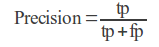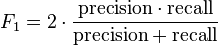# F-ScoresThere has been a lot of talk about F-scores in the chat recently. F-scores are a statistical method for determining accuracy accounting for both precision and for recall or more simply put F-scores are the how HQ determines your accuracy based on what was added and what was missed.

Before we can calculate the final F-score first we must calculate your individual precision and recall. When a player does a cube there are four possible outcomes for every segment in that cube: a true positive result, a false positive result, a false negative result and a true negative result. A true positive (tp) result is when a player adds a segment that should be added. A false positive (fp) is when a player adds a segment that should not be added. A false negative (fn) is when a player misses a segment they should have added and a true negative (tn) is when a player correctly does not add a segment and that segment does not belong. An quick way to remember to which is which is positive means something was added and negative means something wasn’t added and true means it was correctly done and false means it was incorrectly done.  In the figure below you can see an example of false negative and an example of false positive.  In the figure below the green and the red segments are what the player submitted. The yelllow segment was not submitted by the player.The red segment here is a false positive and the yellow segment is a false negative.  The player mistakenly added the red segment when they should have added the yellow segment instead.

This brings us back to precision; precision is how much of a volume was added correctly. For example if Player A has a precision 0.9221 that means about 92% of what Player A added was correct and about 8% of what Player A added should not have been added. To determine a player’s precision we use their true positive (tp) results, correctly added, and their false positive (fp) results, incorrectly added, in this formula:Recall measures how much of the volume was missed. Let’s say Player A has a recall of 0.9409. That means that Player A missed about 6% of the correct segments in the cubes Player A worked on. To determine a player’s recall we use their true positive (tp) results, correctly added, and false negative (fn) results, incorrectly missed, in this formula:Now we would take the results from both of those formulas and plug them into the formula below to get a player’s F-score or another way to look at it is we take the harmonic mean of a player’s precision and recall to get their overall accuracy rating.One question we a get a lot is how do we know what is correct and what isn’t? What is correct is determined by combining the GrimReaper’s corrections with the Eyewirer consensus. If a cube does not have a GrimReaper correction we just use the EyeWirer consensus. We have been able to confirm that the EyeWirer consensus is quite reliable. Once a week our fabulous grad student updates this information for all of our EyeWirers and while a higher F-score implies higher accuracy we currently cannot prove this to be true. It is likely though. In the meantime keep on playing!